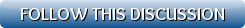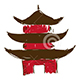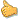[Show all top banners]
 Back to: Kurakani General Discussion Refresh page to view new replies[VIEWED 1610 TIMES] SAVE! for ease of future access.
Brain MalfunctionPosted on 09-25-08 9:42 PM     Reply [Subscribe]
Login in to Rate this Post:     0?QUESTION:1
The sum of the even numbers between 1 and n is 79*80, where n is an odd number, then n=?

QUESTION:2
The price of a bushel of corn is currently \$3.20, and the price of a peck of wheat is \$5.80. The price of corn is increasing at a constant rate of 5x cents per day while the price of wheat is decreasing at a constant rate of cents per day. What is the approximate price when a bushel of corn costs the same amount as a peck of wheat?
(A) \$4.50
(B) \$5.10
(C) \$5.30
(D) \$5.50
(E) \$5.60

QUESTION:3
How many randomly assembled people do u need to have a better than 50% prob. that at least 1 of them was born in a leap year?

QUESTION:4
In a basketball contest, players must make 10 free throws. Assuming a player has 90% chance of making each of his shots, how likely is it that he will make all of his first 10 shots?

QUESTION:5
A and B ran a race of 480 m. In the first heat, A gives B a head start of 48 m and beats him by 1/10th of a minute. In the second heat, A gives B a head start of 144 m and is beaten by 1/30th of a minute. What is B's speed in m/s?
(A) 12
(B) 14
(C) 16
(D) 18
(E) 20

QUESTION:6
AB + CD = AAA, where AB and CD are two-digit numbers and AAA is a three digit number; A, B, C, and D are distinct positive integers. In the addition problem above, what is the value of C?

(A) 1
(B)3
(C) 7
(D) 9
(E) Cannot be determined

QUESTION:7

1. A certain quantity of 40% solution is replaced with 25% solution such that the new concentration is 35%. What is the fraction of the solution that was replaced?
(A) 1/4
(B) 1/3
(C) 1/2
(D) 2/3
(E) Â¾

QUESTION:8

A bag contains 3 red, 4 black and 2 white balls. What is the probability of drawing a red and a white ball in two successive draws, each ball being put back after it is drawn?

(A) 2/27
(B) 1/9
(C) 1/3
(D) 4/27
(E) 2/9

QUESTION:9

What is the least possible distance between a point on the circle x^2 + y^2 = 1 and a point on the line y = 3/4*x - 3?

A) 1.4
B) sqrt(2)
C) 1.7
D) sqrt(3)
E) 2.0

QUESTION:10In the square above, 12w = 3x = 4y. What fractional part of the square is shaded?

A) 2/3

B) 14/25

C) 5/9

D) 11/25

E) 3/7